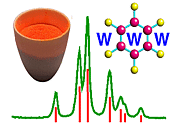Powder Diffraction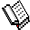Course Material Index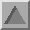Section Index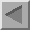Previous Page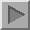Next Page

Powder Diffraction

The kinematic theory of diffraction outlined in this section has been explained with single crystals in mind. It requires only a small step from here to envisage powder diffraction. With single crystals diffraction is, in effect, an arranged event in which the crystal and incident X-ray beam must be configured so that Bragg's law is satisfied and diffraction occurs. If the condition is not satisfied the crystal must be rotated, so that the angle θ becomes equal to that given by Bragg's law, for diffraction to occur.

With powder specimens, such rotation is not essential for diffraction. A powder specimen of a single material would consist of many small crystallites (typically 1 to 10 micron-sized, ideally imperfect crystals) compacted into a powder in which all crystallite orientations are equally likely, the opposite of which is a highly undesirable but common condition known as preferred orientation. If however there are indeed very many crystallites in random orientations then for any given d spacing of the crystal, say d1, there will by chance be a number of crystallites at the correct orientation (angled at θ1 to the incident beam) to satisfy Bragg's law for planes spaced d1 apart:

 λ = 2d1sinθ1
Two such crystallites are indicated in the plane of the figure below, though there will also be many other crystallites angled "azimuthally" at θ1 so that diffraction also occurs out of the plane of the figure; altogether a cone of diffracted rays, with a semi-vertex angle of 2θ1, (remember θ is the angle between the incident beam and the d-planes whereas 2θ is the angle between the incident and diffracted beams) is produced as illustrated in red.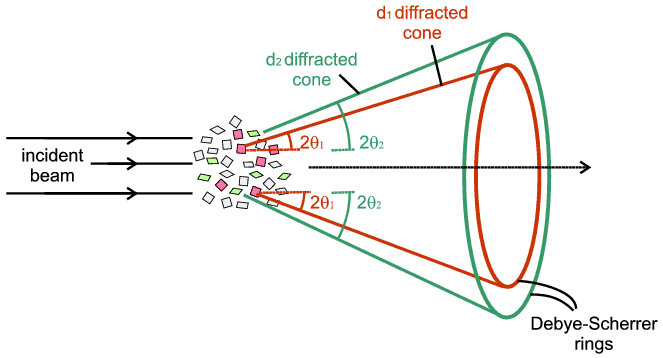Simultaneously there will also by chance be a number of crystallites at the correct orientation (angled at θ2 to the incident beam) to satisfy Bragg's law for planes spaced d2 apart:

 λ = 2d2 sinθ2
This will produce another cone of diffracted rays (semi-vertex angle 2θ2) illustrated in green, and so on for d3, d4 etc. These cones would intersect a flat photographic plate as circles, known as Debye-Scherrer rings after the early powder diffraction camera invented by Debye, Scherrer and Hull.

The intensity of these diffraction objects will be the centre of our attention next since the intention of structural crystallography is to link atomic structure to diffraction intensities via the theory of kinematic diffraction as expressed in the structure factor equation (see previous page in Diffraction I). In the case illustrated the experimentally measured intensities would be obtained from the rings of darkened emulsion on the photographic film, though equally it could be from an electronic X-ray detector scanning through the same region. Whichever experimental method is used, the actual intensities measured will depend on many experimental factors such as the state of the specimen, exposure time and the precise diffraction geometry. The less obvious of these factors constitute a standard set of "corrections" (as they are often referred to, though it is not a good term) that have to be made to the experimental data (the intensities) before they can be used in the structure factor equation to yield a reliable result for the atomic structure. These "corrections" will also be dealt with in this section.Course Material IndexSection IndexPrevious PageNext Page
 © Copyright 1997-2006.  Birkbeck College, University of London. Author(s): Paul Barnes Simon Jacques Martin Vickers